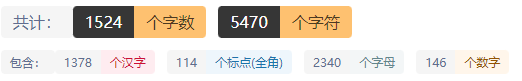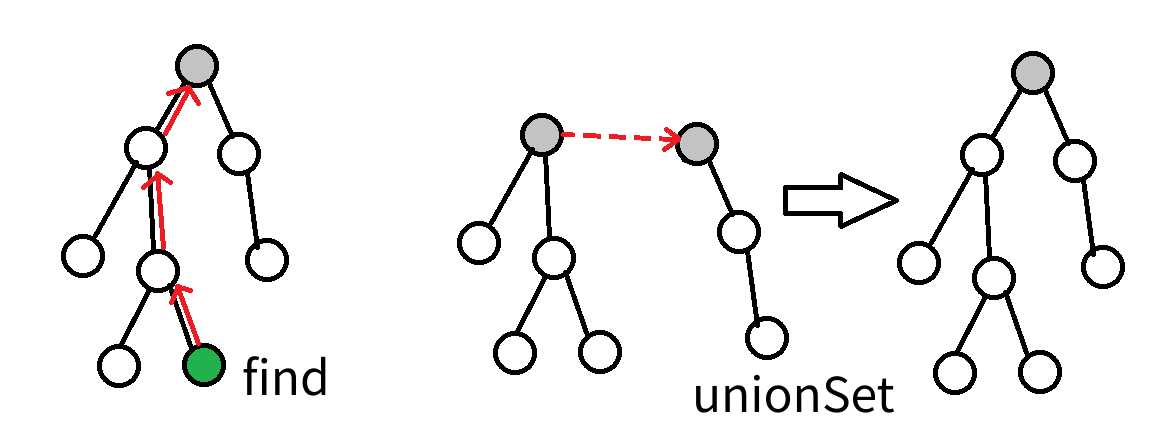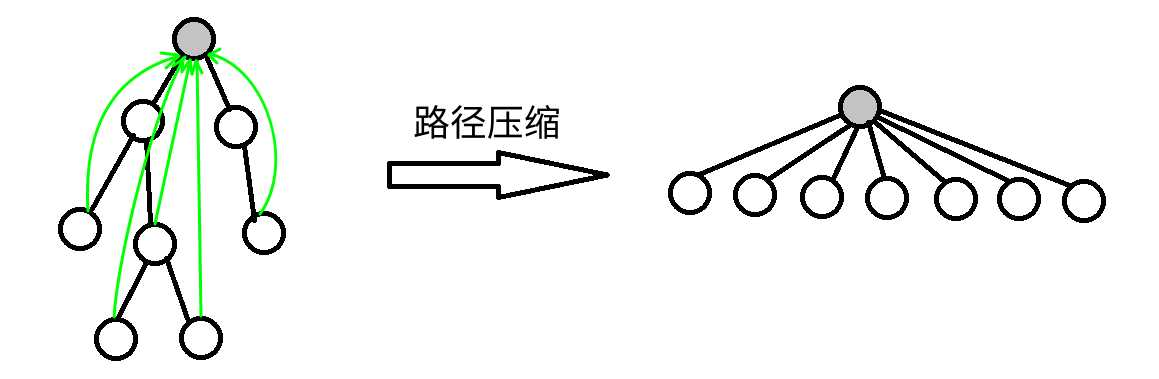# 并查集¶

## 一、并查集简介¶

1.查找（find）：确定某个元素处于哪个子集

2.合并（merge）：将两个子集合并成一个集合

## 二、初始化¶

void init(int n) {
for(int i = 1;i <= n; ++i) fa[i] = i;
}


## 三、查找¶

int find(int x) {
whille(x != fa[x]) x = fa[x];
return x;
}


int find(int x) {
if(x == fa[x]) return x;//如果x的父亲就是x那么就是找到了x的祖先节点
return find(x);//递归返回
}


## 四、路径压缩¶int find(int x) {
int t = x;
while(t != fa[t]) t = fa[t];//直接查找x的祖先节点
while(x != fa[x]) {
int temp = fa[x];
fa[x] = t;//从下到上更新fa[x]的值为x的祖先节点的值
x = temp;
}
return x;
}


int find(int x) {
if(x != fa[x]) fa[x] = find(x);//如果x不是自己的父亲节点那么就找到x的父亲节点，一路上路径压缩
return fa[x];
}


## 五、合并¶

void merge(int a,int b) {
a = find(a);
b = find(b);
if(a != b) fa[b] = a;//如果两个元素不相等就让一个元素成为另一个元素的父节点
}


## 七、练习题目¶

https://www.luogu.com.cn/problem/P1551 P1551 亲戚
https://www.luogu.com.cn/problem/P1536 P1536 村村通
https://www.luogu.com.cn/problem/P1525 P1525 [NOIP2010 提高组] 关押罪犯（种类并查集）
https://www.luogu.com.cn/problem/P1621 P1621 集合
https://www.luogu.com.cn/problem/P1892 P1892 [BOI2003]团伙
https://www.luogu.com.cn/problem/P1955 P1955 [NOI2015] 程序自动分析(这个有趣)
https://www.luogu.com.cn/problem/P2814 P2814 家谱(这个简单，不过字符串处理一下)
https://vjudge.net/contest/445444 这个并查集题单还是挺有趣的
http://acm.hdu.edu.cn/showproblem.php?pid=3038 How Many Answers Are Wrong（这是一个带权并查集，感兴趣的可以试试）